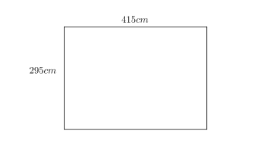Courses
Courses for Kids
Free study material
Free LIVE classes
More# Amanda’s bedroom is a rectangle 415cm long by 295cm wide.(a) Work out the area of Amanda’s bedroom. Give your answer in square meters $\left( {{m}^{2}}\right)$ . Show how to use estimation to check your answer.A carpet costs $\$ 24 $per square meter. It is sold in whole numbers of square meters.(b) How much will it cost Amanda to buy carpet for her bedroom floor? Show how to use inverseoperations to check your answer. AnswerVerified 335.1k+ views Hint: We have to find the area of Amanda’s bedroom which is in the shape of a rectangle in square meters.To find the area in square meters we convert the concert length and width into meters first and then calculate the area of the rectangle by using formula length × width. After calculating the area we convert it to the nearby whole number since carpet is sold in whole numbers of square meters. We then calculate the total cost of carpet by multiplying it with total area required. Complete step-by-step answer: According to the problem, we have a rectangular bedroom with 415cm long by 295cm wide. We know that$ 1cm=0.01m $. Length of bedroom =$ 415 \times 0.01=4.15m $. Width of bedroom =$ 295 \times 0.01=2.95m $.We need to find the area of the rectangular bedroom in square meters. We know that the area of a rectangle with length ‘l’ and width ‘w’ is given by$ l\times w $. Let us assume the area of the bedroom to be A.$ A=4.15\times 2.95 $A = 12.2425 square meters. We have a cost of carpet$ 24 $per square meter as given from the problem. We can also observe that the carpet is sold in a whole number of square meters. So, we need to convert the area to the nearest whole number. We assume an area as 12 square meters. ∴$ A=12{{m}^{2}} $Now we find the total cost required to buy carpet of$ 12{{m}^{2}} $. We know that total cost of carpet = cost per square meters of area × Total area of carpet. Total cost = 24 × 12 Total cost =$ \$288.$
$\$ 288. $to buy a carpet for her bedroom floor Area of bed room 12.2425 square meters and total cost incurred is$ \$288$